Question

# The force P is applied to (a) the 63-lb block and (b) the 89-lb block. For...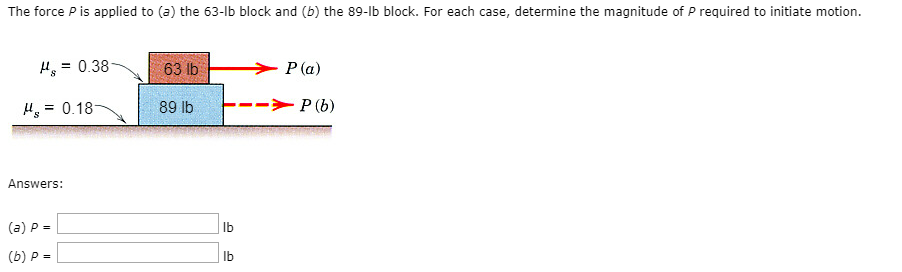The force P is applied to (a) the 63-lb block and (b) the 89-lb block. For each case, determine the magnitude of P required to initiate motion. ?.-0.38 63 lb -P (a) ?,-0.18 89 lb -- Answers: (a) P- lb (b) P- lb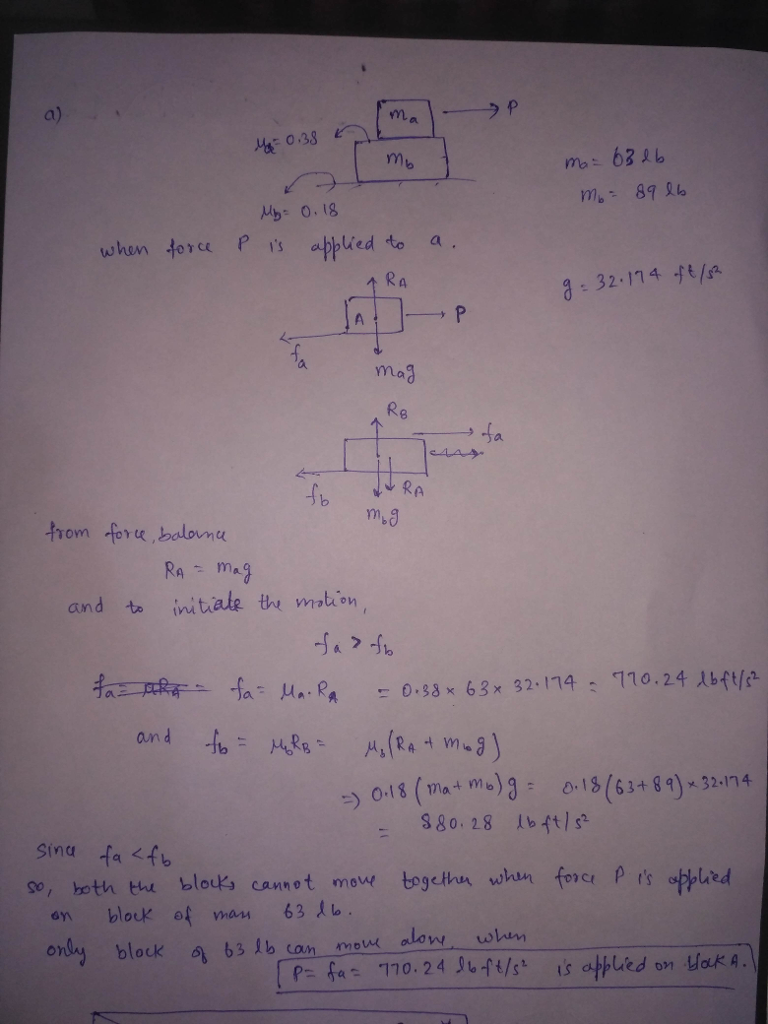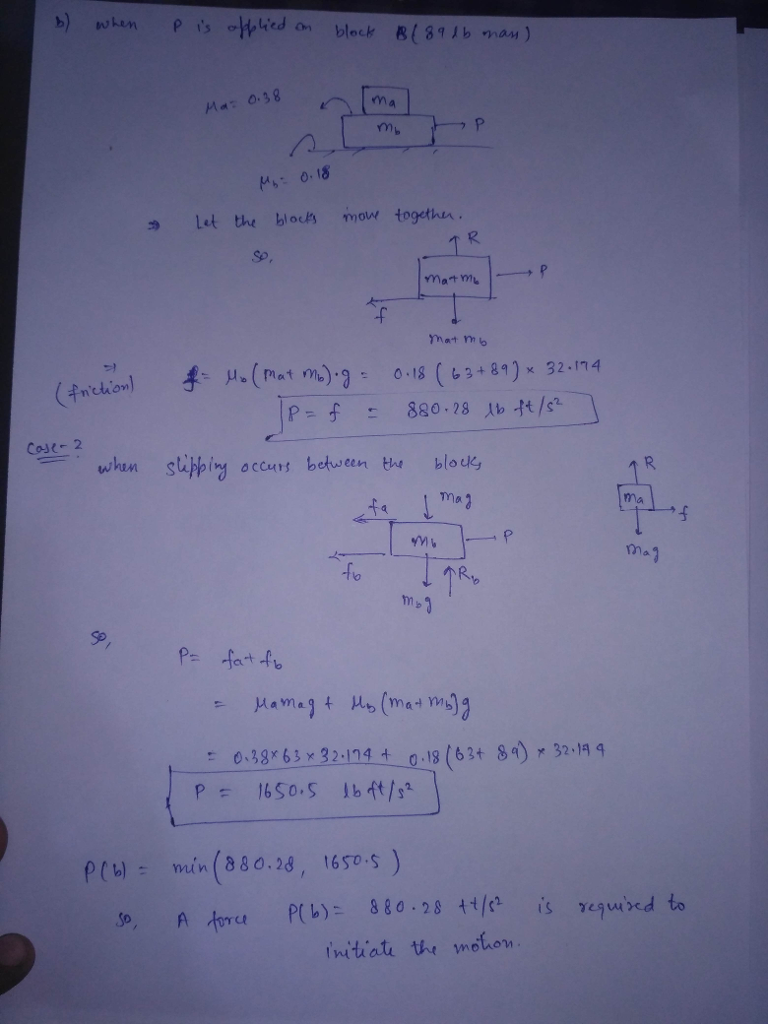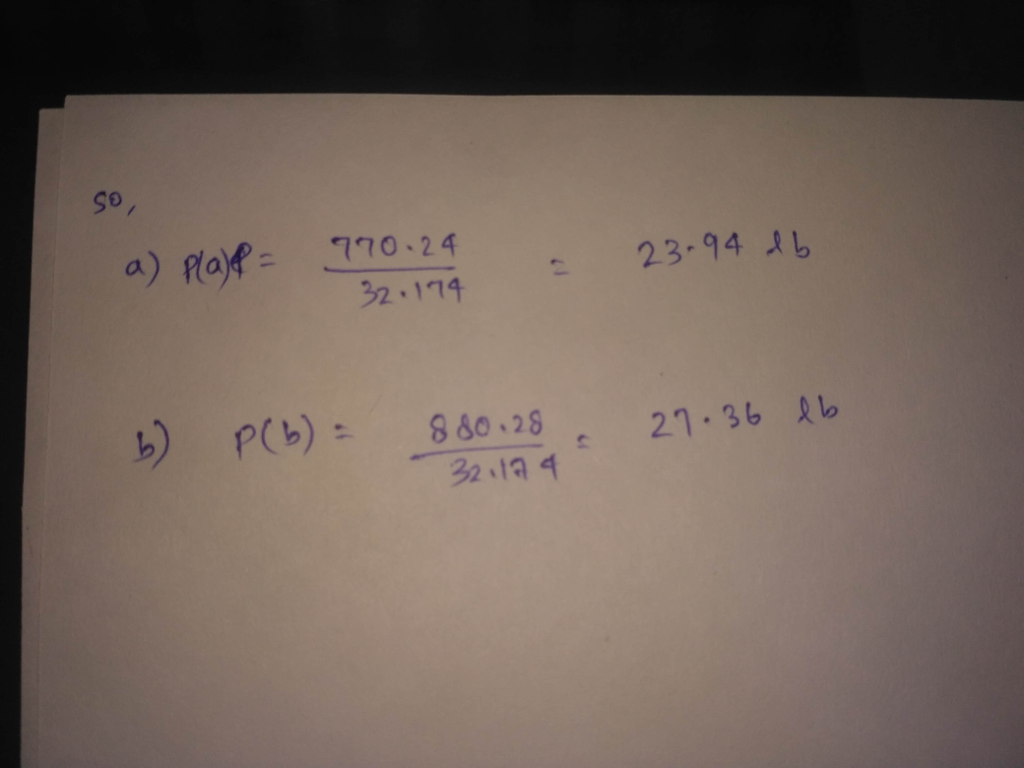#### Earn Coins

Coins can be redeemed for fabulous gifts.

Similar Homework Help Questions
• ### The force P is applied to the 42-kg block when it is at rest. Determine the...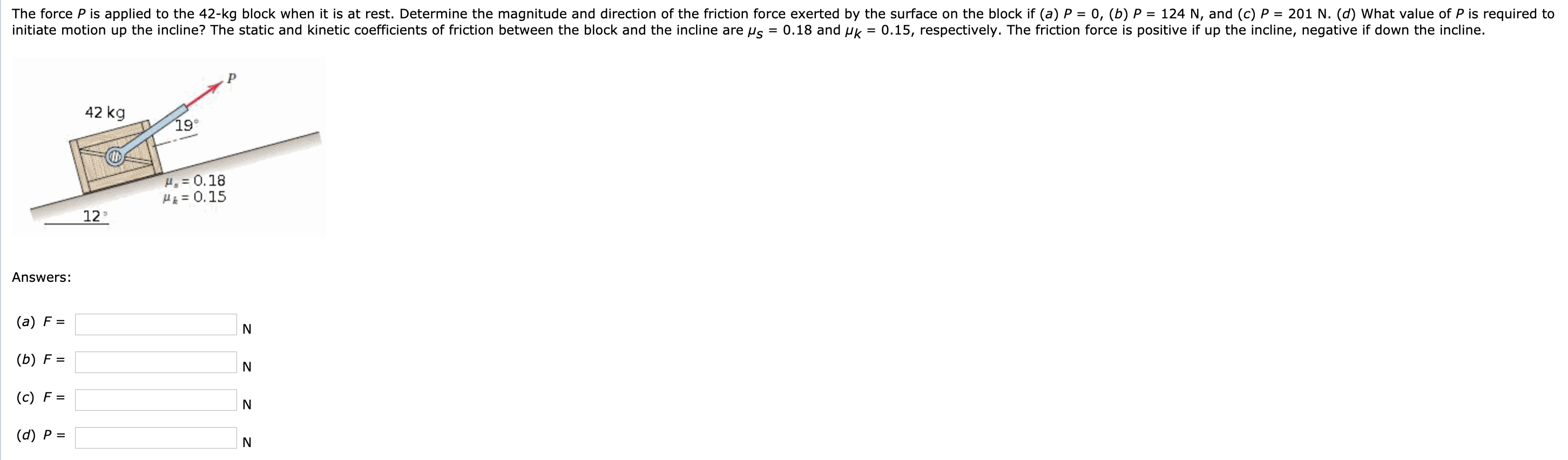The force P is applied to the 42-kg block when it is at rest. Determine the magnitude and direction of the friction force exerted by the surface on the block if (a) P = 0, (b) P = 124 N, and (c) P = 201 N. (d) What value of P is required to initiate motion up the incline? The static and kinetic coefficients of friction between the block and the incline are us = 0.18 and uk = 0.15,...

• ### The force Pis applied to the 60-kg block when it is at rest. Determine the magnitude...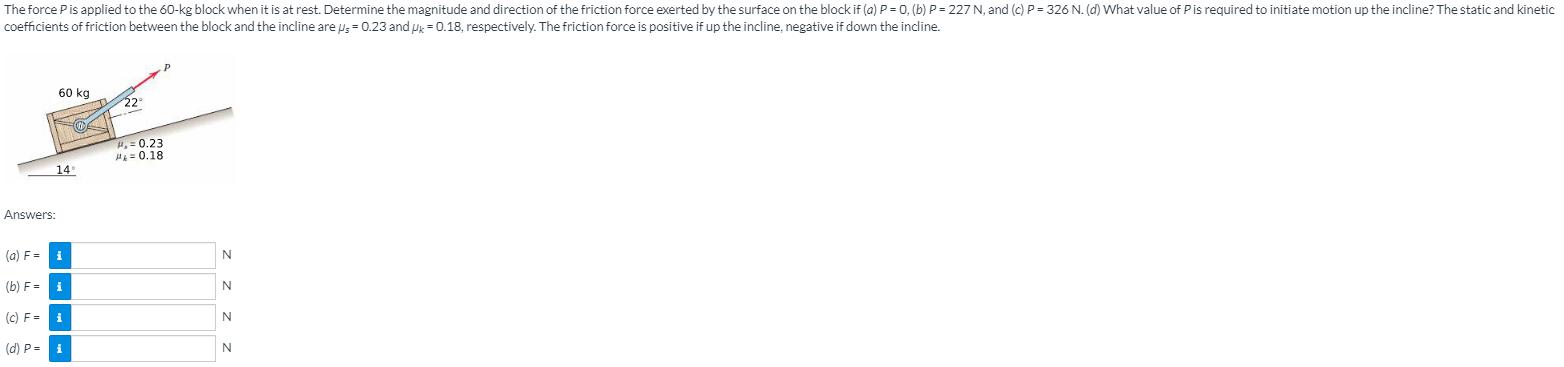The force Pis applied to the 60-kg block when it is at rest. Determine the magnitude and direction of the friction force exerted by the surface on the block if (a) P = 0. (b) P=227 N, and (c) P= 326 N. (d) What value of Pis required to initiate motion up the incline? The static and kinetic coefficients of friction between the block and the incline are s = 0.23 and Uk = 0.18, respectively. The friction force is...

• ### The force P is applied to the 67-kg block when it is at rest. Determine the...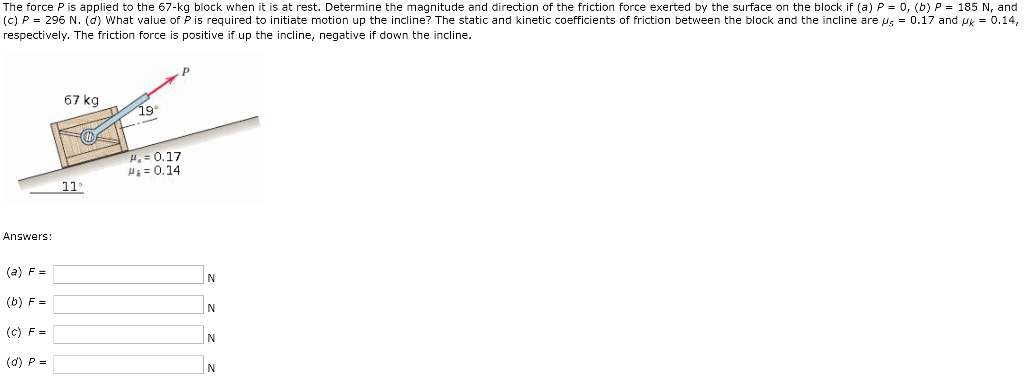The force P is applied to the 67-kg block when it is at rest. Determine the magnitude and direction of the friction force exerted by the surface on the block if (a) P = 0, (b) P 185 N, and (c) P 296 N. (d) What value of P is required to initiate motion up the incline? The static and kinetic coefficients of friction between the block and the incline are us 0.17 and u 0.14 respectively. The friction force...

• ### The force P is applied to the 49-kg block when it is at rest. Determine the...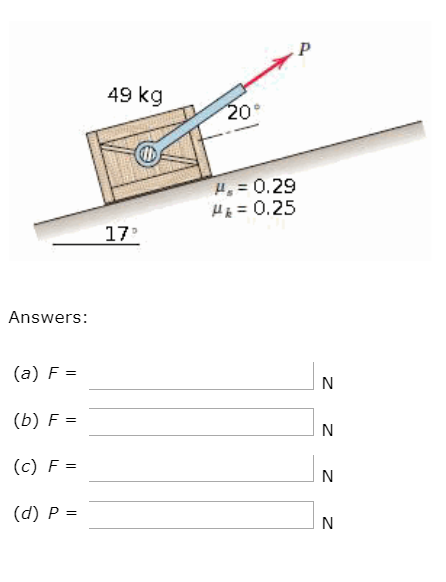The force P is applied to the 49-kg block when it is at rest. Determine the magnitude and direction of the friction force exerted by the surface on the block if (a) P = 0, (b) P = 219 N, and (c) P = 311 N. (d) What value of P is required to initiate motion up the incline? The static and kinetic coefficients of friction between the block and the incline are μs = 0.29 and μk = 0.25,...

• ### P3 Determine the magnitude P of the horizontal force required to initiate motion of the block of ...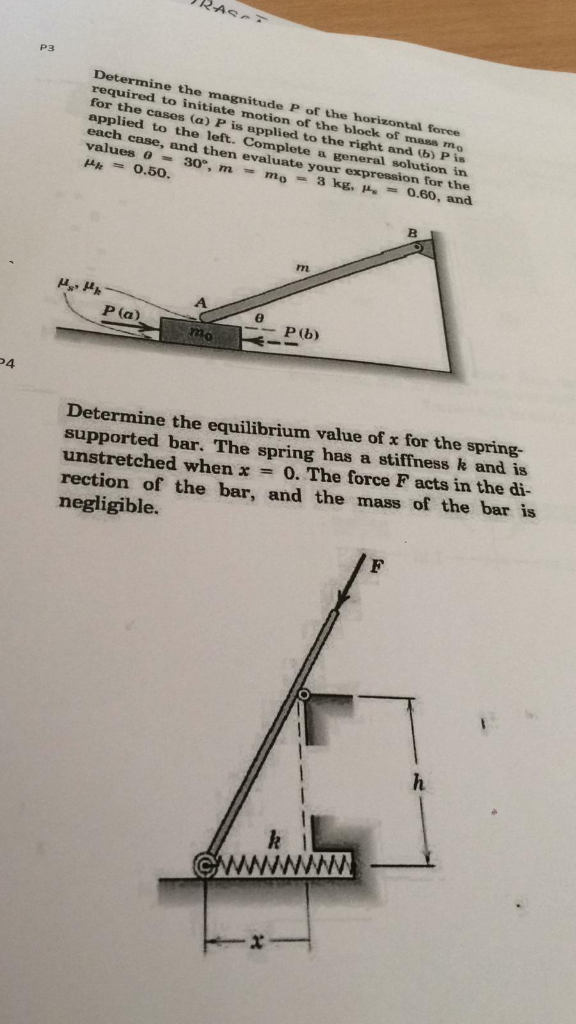P4 asap please P3 Determine the magnitude P of the horizontal force required to initiate motion of the block of mass mo for the cases (a) P is applied to the right and b) Pis applied to the left. Complete a general solution each case, and then evaluate your exp in ression for the μ'. 0.50. mo 3 kg, 0.60, and rm P(a) P (b) 4 Determine the equilibrium value of x for the spring- supported bar. The spring has...

• ### please explain steps thanks The force P is applied to the 43-kg block when it is...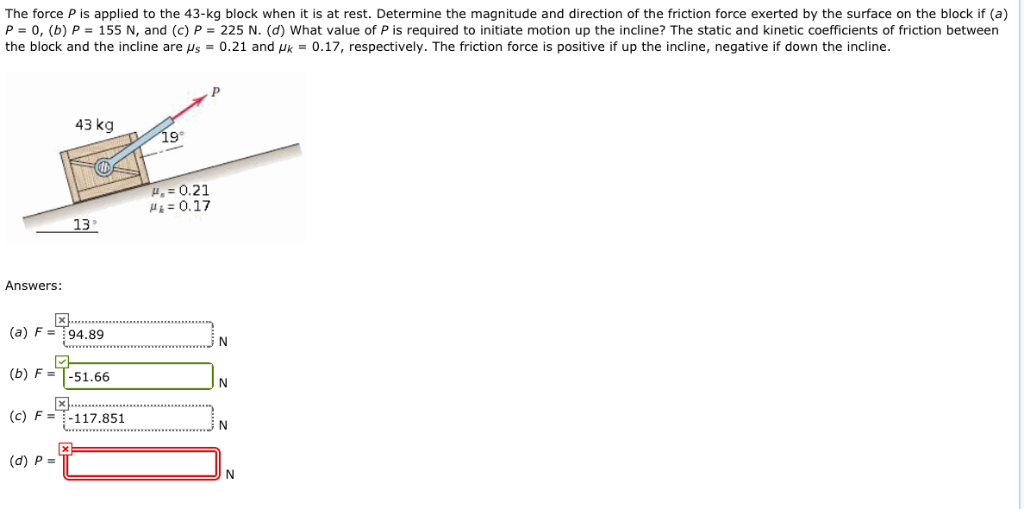please explain steps thanks The force P is applied to the 43-kg block when it is at rest. Determine the magnitude and direction of the friction force exerted by the surface on the block if (a) P = 0 b) P-155 N, and c) P = 225 N d what value of P is required to initiate motion up the incline? The static and ki etic coefficients of friction between the block and the incline are μs = 0.21 and...

• ### Chapter S6, Problem S6/003 GO Tutorial The force P is applied to the 37-kg block when...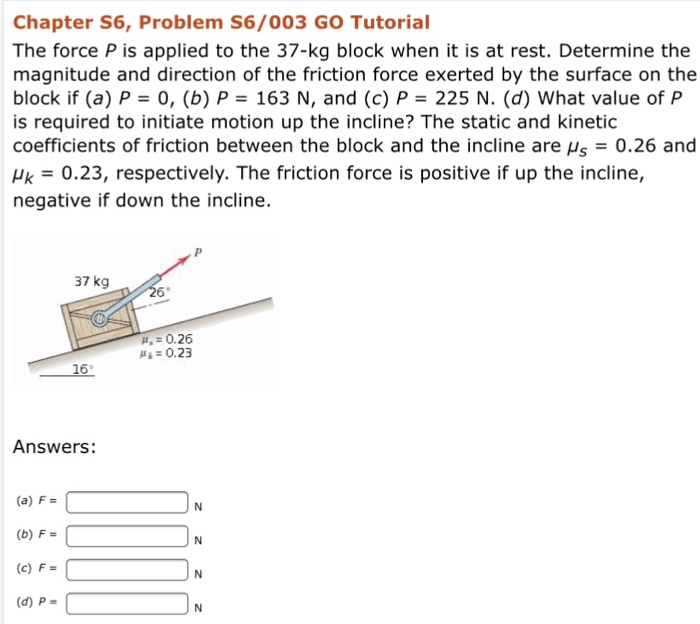Chapter S6, Problem S6/003 GO Tutorial The force P is applied to the 37-kg block when it is at rest. Determine the magnitude and direction of the friction force exerted by the surface on the block if (a) P 0, (b) P 163 N, and (c) P 225 N. (d) What value of P is required to initiate motion up the incline? The static and kinetic coefficients of friction between the block and the incline are Hs0.26 and k 0.23,...

• ### A 200 lb block resting on a horizontal plane. Determine the magnitude of the force P...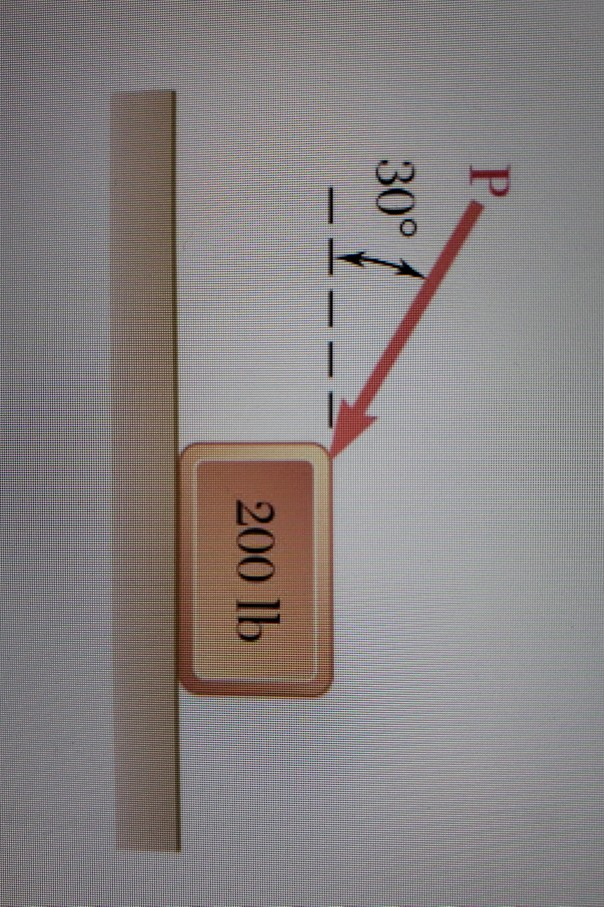A 200 lb block resting on a horizontal plane. Determine the magnitude of the force P that is required to give the block an acceleration of 10 feet / s2 to the right. The coefficient of kinetic friction between the block and the plane is π 0.30. 30° - - - 200 lb

• ### The 88-lb force P is applied to the 200-lb crate, which is stationary before the force...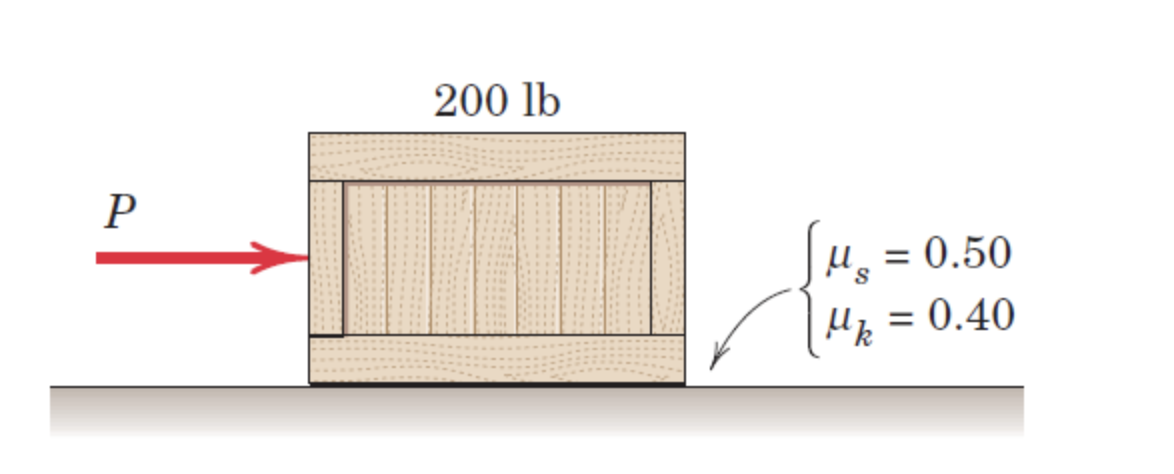The 88-lb force P is applied to the 200-lb crate, which is stationary before the force is applied. Determine the magnitude of the friction force F exerted by the horizontal force on the crate. Please show complete solution. 200 lb Р ſus = = 0.50 r Up = 0.40

• ### The 83-1b force P is applied to the 170-lb crate, which is stationary before the force...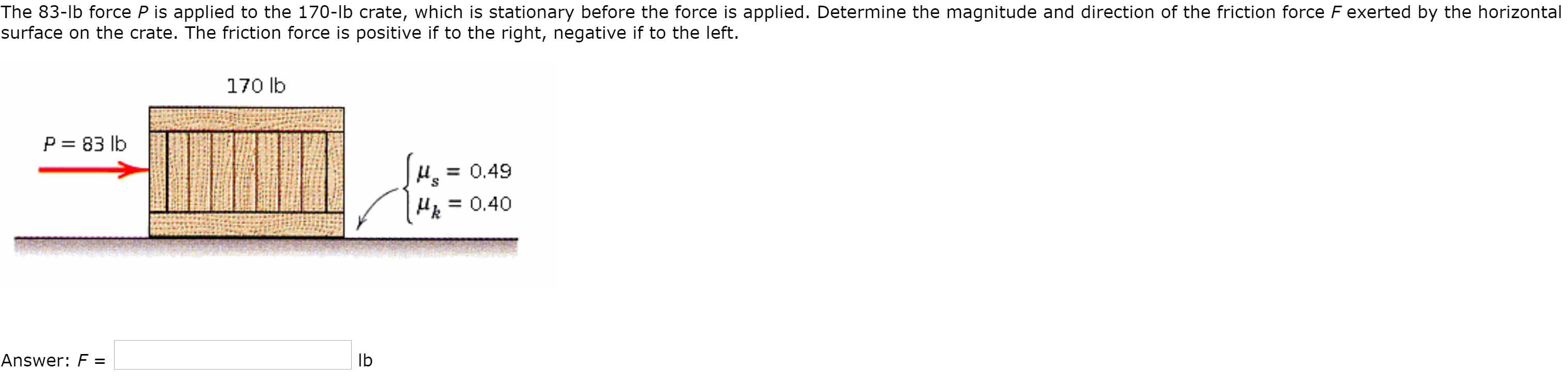The 83-1b force P is applied to the 170-lb crate, which is stationary before the force is applied. Determine the magnitude and direction of the friction force F exerted by the horizontal surface on the crate. The friction force is positive if to the right, negative if to the left. 170 lb P= 83 lb up = 0.49 MR = 0.40 Answer: F =